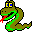#Boost C++ Libraries

...one of the most highly regarded and expertly designed C++ library projects in the world.#Bellman Ford Visitor Concept

This concept defines the visitor interface for bellman_ford_shortest_paths(). Users can define a class with the Bellman Ford Visitor interface and pass and object of the class to bellman_ford_shortest_paths(), thereby augmenting the actions taken during the graph search.

### Refinement of

Copy Constructible (copying a visitor should be a lightweight operation).

### Notation

 V A type that is a model of Bellman Ford Visitor. vis An object of type V. G A type that is a model of Graph. g An object of type G. e An object of type boost::graph_traits::edge_descriptor. s,u An object of type boost::graph_traits::vertex_descriptor.

none

### Valid Expressions

NameExpressionReturn TypeDescription
Examine Edge vis.examine_edge(e, g) void This is invoked on every edge in the graph num_vertices(g) times.
Edge Relaxed vis.edge_relaxed(e, g) void Upon examination, if the following condition holds then the edge is relaxed (its distance is reduced), and this method is invoked.
tie(u,v) = incident(e, g);
D d_u = get(d, u), d_v = get(d, v);
W w_e = get(w, e);
assert(compare(combine(d_u, w_e), d_v));
Edge Not Relaxed edge_not_relaxed(e, g) void Upon examination, if the edge is not relaxed (see above) then this method is invoked.
Edge Minimized vis.edge_minimized(e, g) void After num_vertices(g) iterations through the edge set of the graph are completed, one last iteration is made to test whether each edge was minimized. If the edge is minimized then this function is invoked.
Edge Not Minimized edge_not_minimized(e, g) void If the edge is not minimized, this function is invoked. This happens when there is a negative cycle in the graph.

### Python

To implement a model of the BellmanFordVisitor concept in Python, create a new class that derives from the BellmanFordVisitor type of the graph, which will be named GraphType.BellmanFordVisitor. The events and syntax are the same as with visitors in C++. Here is an example for the Python bgl.Graph graph type:
```class count_tree_edges_bellman_ford_visitor(bgl.Graph.BellmanFordVisitor):
def __init__(self, name_map):
bgl.Graph.BellmanFordVisitor.__init__(self)
self.name_map = name_map

def edge_relaxed(self, e, g):
(u, v) = (g.source(e), g.target(e))
print "Relaxed edge ",
print self.name_map[u],
print " -> ",
print self.name_map[v]
```

 Copyright © 2000-2001 Jeremy Siek, Indiana University (jsiek@osl.iu.edu) Lie-Quan Lee, Indiana University (llee@cs.indiana.edu) Andrew Lumsdaine, Indiana University (lums@osl.iu.edu)